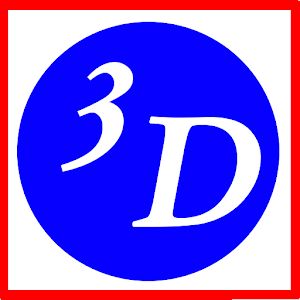# Analytic GeometryRating: 3.7/5

Introduction

The programme “Analytic Geometry” for Android™ is a flexible system designed for representing geometrical objects (on an OpenGL basis) in a rotatable 3d coordinate system, using parallel or central projection.

The programme supports the following objects: Point, vector, line segment, straight line, circle, ellipse, rectangular solid, tetrahedron, pyramid, prism, sphere, cone, cylinder and torus.

Distances and intersections can be calculated for the most often used combinations of objects. The objects resulting from these calculations are added to the scene and are adapted automatically, when one of the initial objects changes.

Also the elementary level of calculations is included. 2 vectors can be tested for collinearity or 3 vectors for linear indepency. In addition linear combinations of vectors can be drawn.

As a bonus, the graph of a function of two variables and that of a function in parametric form can be added to the scene.

There is the option to show an object’s data in a portion of the screen.

Of course, the whole scene can be saved and reloaded.

Those of you interested in mathematics outside the educational sector, too, will enjoy the capabilities of the programme.

The emphasis is on a flexible representation of the scene as well as on the calculations of distances and intersections.

We have added a learning aid, that introduces the basic definitions and concepts of analytic geometry.